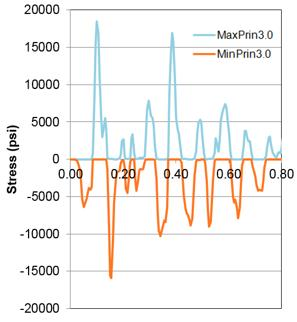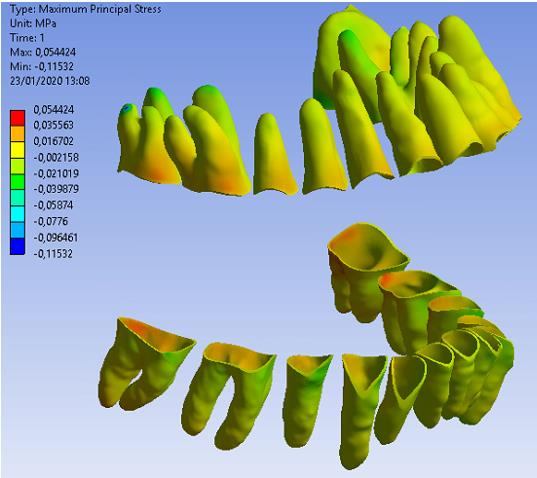# Von-Mises Stress

Hi,

my question is about the Equivalent Von-Mises stress output. I would like to get a clarification about its interpretation to check for validation of the model. I know it is the square root of the stress along the principal axes, thus it is always positive.

If I have a model subjucted to a force vector is it right to assume that the red coloured areas of the Equivalent stress plot, which are of higher magnitude) refer to a compression? Or it includes also the tension? This because looking at the reaction forces the output doesn't behave as the force applied requires.

Any response will be appreciated.

Thanks.

• With Equivalent von-Mises Stress, you can't tell if it is tension or compression.

Plot Maximum Principal Stress to see the stress that is most tensile (or zero).

Plot Minimum Principal Stress to see the stress that is most compressive (or zero).

Below is a plot of those two stresses vs time at a point on a part that was vibrating.The point was going from tension to compression as the part bounced around.

• edited February 2020

ok, thank you Peter! Few questions arise now:

- why do I have positive and negative values about maximum principal stress? The same occur if I evaluate the minimum principal stress. What does it mean?

- What does the middle principal stress mean?

• Show an image of the Maximum Principal Stress plot.

It is easier to understand the concepts for a 2D state of stress.  Read up on Mohr's circle.

If all components of stress are compressive, for example with hydrostatic pressure, then the Maximum Principal Stress can be negative.

• edited February 2020

Hi,

this is the plot of max principal stresshere a force is applied about at the height of the 4 body (from the left) and points downward and forward, thus it makes sense that anteriorly bodies exhibit compression. However, the plot is about maximum principal stress, not minimum. Here not all the components are compressed, some parts are tensioned (positive value).

Thank you!